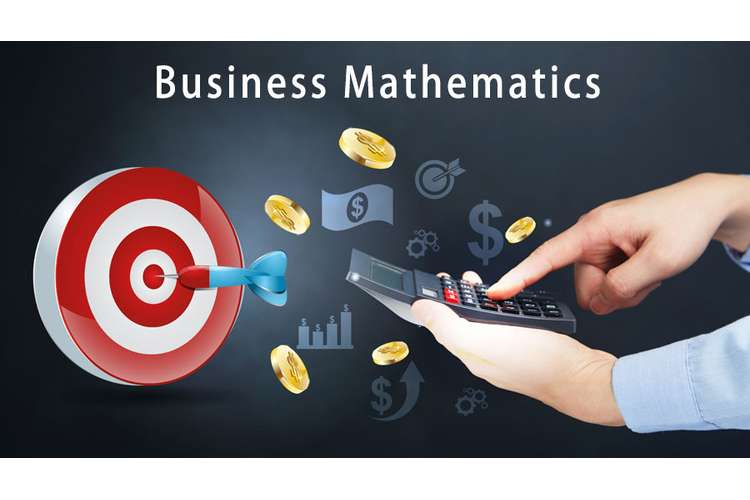## A. Frequency distribution table (examination score)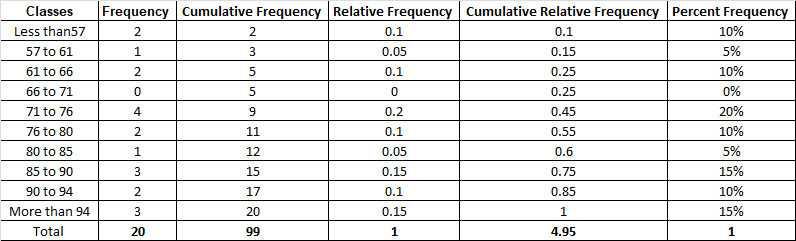B. Histogram (examination score)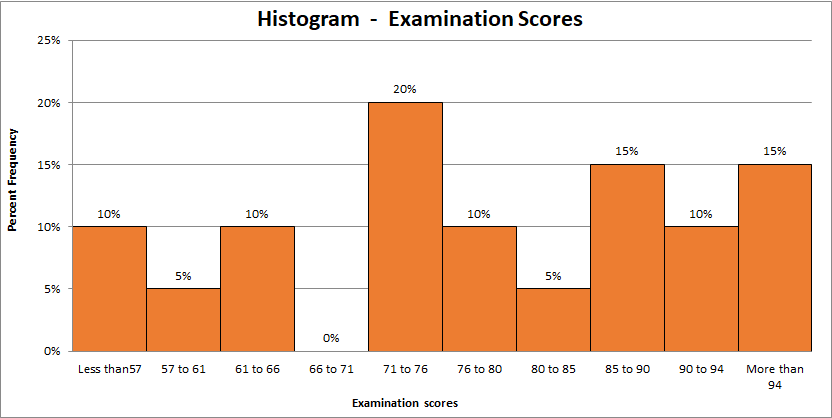The histogram shape is not bell shaped which indicates that distribution is not- normal. Further, there is significantly high variation is observed in the data which is evident from the presence of negative skew (Harmon, 2016). Therefore, the conclusion can be made that examination scores does not follow normal distribution.

### Question 2

Supply (y) of the product is in the capacity of dependent variable and unit price (x) of the product is in the capacity of independent variable.

ANOVA TABLE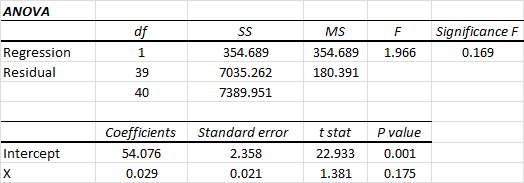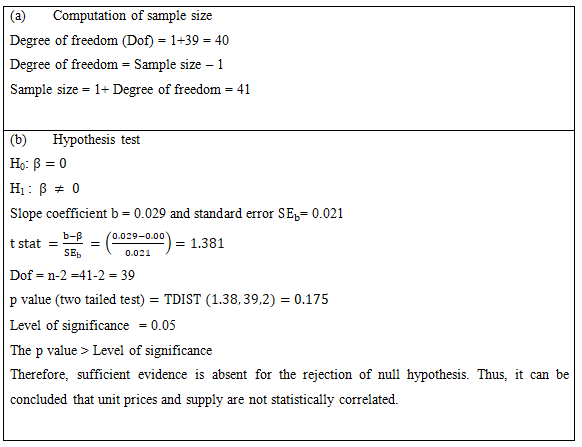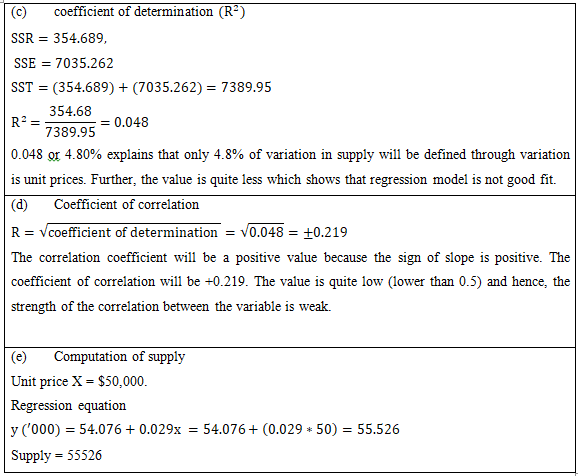### ANOVA TABLE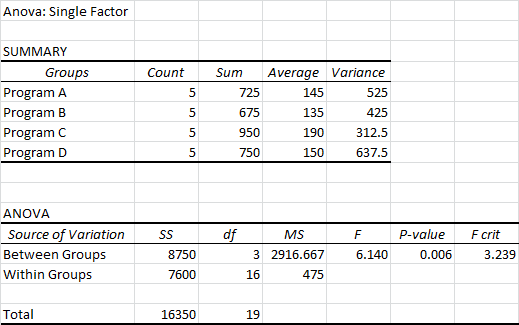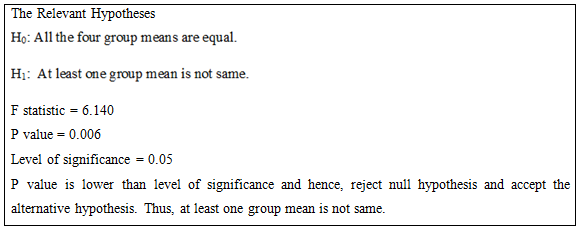### A. Regression output by considering sales in the capacity of dependent variable and price and advertising expenses in the capacity of independent variables.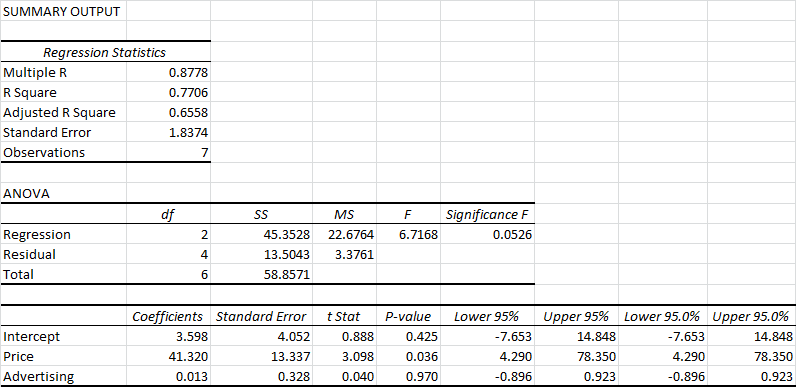Least square regression line equation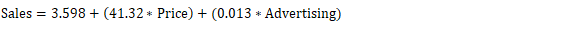B. Hypotheses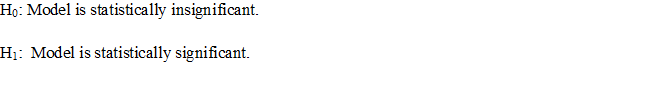From ANOVA TABLE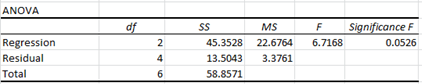Given level of significance = 0.10

The F stat = 6.7168

Significance F = 0.0526

The significance F is lesser than level of significance. As a result, reject null hypothesis and accept alternative hypothesis(Flick, 2015). Thus, model is considered to be statistically significant.

C. The hypothesis for testing the slope significance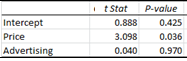Level of significance = 0.10

For Price: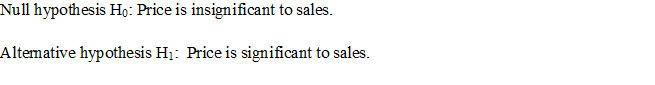From the table shown above, it would be fair to conclude that p value for price is lower than level of significance (0.036<0.10). Thus, reject null and accept alternative hypothesis. Price is significant to sale.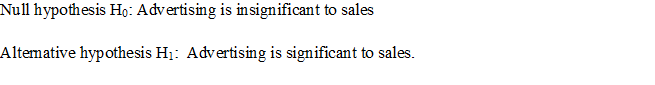From the table shown above, it would be fair to conclude that p value for advertising is greater than level of significance (0.97<0.10). Thus, cannot reject null hypothesis. Advertising is not statistically significant to sale (Hair, et.al., 2016).

D. Regression model after removing the advertising expenditure variable which is insignificant.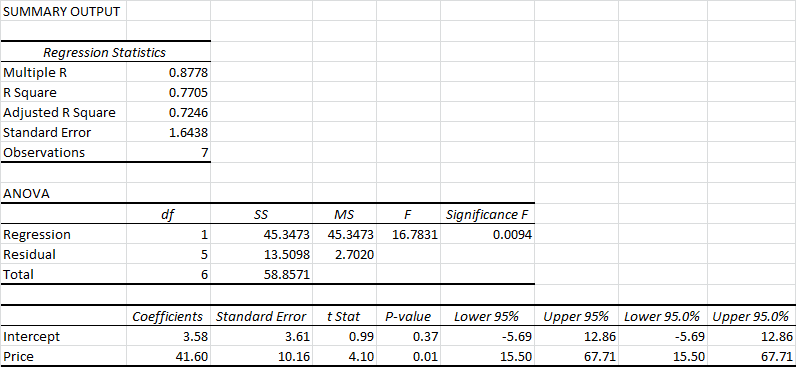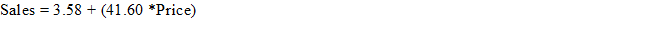Least square regression line equation

E. “The key understating that can be drawn for the slope coefficient is that whenpriceincreased by \$1, then sales will also increase by 41.60 units.”

#### Reference

1. Flick, U. (2015) Introducing research methodology: A beginner's guide to doing a research project.4th ed. New York: Sage Publications.
2. Hair, J. F., Wolfinbarger, M., Money, A. H., Samouel, P., and Page, M. J. (2016) Essentials of business research methods.2nd ed. New York: Routledge.
3.  Harmon, M. (2016)Hypothesis Testing in Excel - The Excel Statistical Master.7th ed. Florida: Mark Harmon.